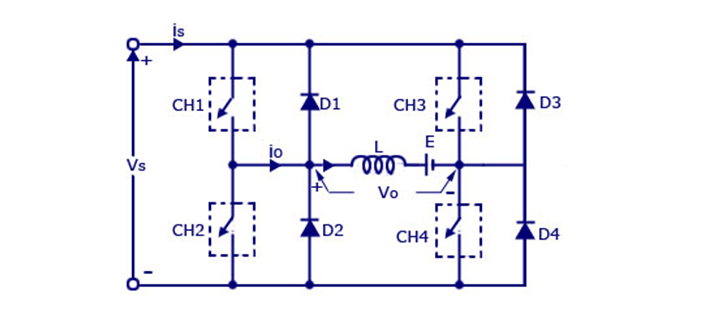# Chopper

Jun 12 • General, Notes • 2597 Views • No Comments on Chopper

1. CHOPPER

1.1 DEFINITION OF CHOPPER

A chopper is a device that is static in nature and which is used to obtain a dc voltage(variable)   from a dc voltage (constant) source. A chopper is otherwise also known as dc to dc converter. They can be used in cars, vehicles operated through batteries , motor control, control of large number of dc motors, etc. They can also be used in braking of dc motors regeneratively  to get back the voltage regulators and also the supply
Choppers are divided into two types:
Step down
Step up

1.2 In step down choppers, the o/p voltage is always small  than the
i/p voltage whereas in step up choppers o/p voltage will be
always greater than the input voltage.

2. Principle in step down chopper

There is a Thyristor in the circuit which acts as a switch. while the thyristor is ON the the voltage supplied is visible across the load and while the thyristor is OFF, the voltage in the load is zero.When the chopper is ON the supply gets connected to the load. This leads to the flow of current from the supply to the load. When the chopper is OFF the current in the load flow s in the same direction across the free-wheeling diode because of the energy stored in the inductor L.

The current in the load can be continuous or can also be discontinuous depending on the values of L. For the current operating continuously the load current always varies between I(max) and I(min).

There also exists step down choppers that obtain a load voltage V0 that is always greater than the input voltage V. Depending upon the requirement of o/p current and voltage the values of L and C are chosen. The inductor L gets connected across the load while the chopper is ON. During this process the current in the inductor rises and the inductor stores the energy while the chopper is ON. While the chopper is OFF the current in the inductor is allowed to flow through a diode. After that the current decreases and the polarity of the induced EMF is reversed.

choppers are also classified according to class.

class Aclass BQ1> Give the equation on which a chopper operates?

ans>

f(h) = f(1)*(k*q±1)±6*n*f(o),

where

f(h) = harmonic frequency imposed on the AC line

k and n = integers

q = pulse number (6, 12 . . .)

f(o) = output frequency of the CCV

Equation’s 1st term represents the pulse number converter harmonic components starting with six-pulse configuration.

GATE Syllabus-

IES syllabus-